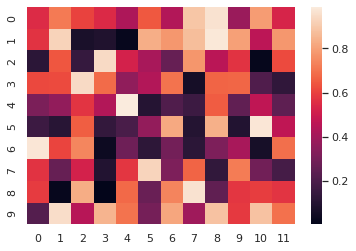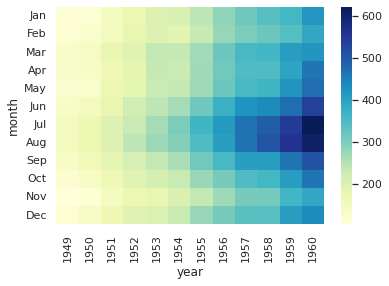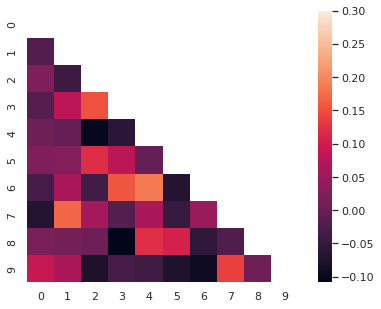To plot heatmap(heat map) in Python we can use different libraries like:

• seaborn
• matplotlib

or combination or them.

## Steps

• Import libraries - matplotlib, seaborn
• Select data to be plot
• create pivot table
• Plot the heat map
• select heat map type
• select color scheme

Note: If you like to plot Pandas DataFrame as a heatmap you can check:
How to Display Pandas DataFrame As a Heatmap

## Example 1 - Heatmap

### Data

Data will be created by `np.random.rand(10, 12)`. It is arrays of random numbers:

``````array([[0.5488135 , 0.71518937, 0.60276338, 0.54488318, 0.4236548 ,
0.64589411, 0.43758721, 0.891773  , 0.96366276, 0.38344152,
0.79172504, 0.52889492],
[0.56804456, 0.92559664, 0.07103606, 0.0871293 , 0.0202184 ,
0.83261985, 0.77815675, 0.87001215, 0.97861834, 0.79915856,
0.46147936, 0.78052918],
``````

### Code

So to plot multiple plots with matplotlib we need to provide the `plt.figure()`

``````import numpy as np; np.random.seed(0)
import matplotlib.pyplot as plt
import seaborn as sns; sns.set_theme()

uniform_data = np.random.rand(10, 12)

ax = sns.heatmap(uniform_data)
``````

### Output## Example 2 - Python heatmap colors

### Data

For this example we will use data coming with the seaborn library:

year 1949 1950 1951 1952 1953 1954 1955 1956 1957 1958 1959 1960
month
Jan 112 115 145 171 196 204 242 284 315 340 360 417
Feb 118 126 150 180 196 188 233 277 301 318 342 391
Mar 132 141 178 193 236 235 267 317 356 362 406 419
Apr 129 135 163 181 235 227 269 313 348 348 396 461
May 121 125 172 183 229 234 270 318 355 363 420 472

### Code

First we load the dataset and then do a pivot table on columns:

• month - index
• year - columns
• passengers - values

To change colors of heatmap in Python we can use parameter: `cmap="YlGnBu"`

``````flights = sns.load_dataset("flights")
flights = flights.pivot("month", "year", "passengers")
ax = sns.heatmap(flights, cmap="YlGnBu")
``````

If you like to find more Python and matplotlib colors please check: Full List of Named Colors in Pandas and Python

### Output## Example 3 - Correlogram

### Data

Again we are using random numbers:

``````array([[ 1.        ,  0.08615821, -0.02380759,  0.01254937, -0.09259778,
-0.06358862, -0.01470216, -0.07846244, -0.02754994,  0.00490301],
[ 0.08615821,  1.        ,  0.18129382, -0.13650656,  0.05585866,
0.02908082, -0.10838342,  0.04390444, -0.03423537,  0.02155898],
[-0.02380759,  0.18129382,  1.        , -0.0554918 ,  0.01511297,
0.09564635, -0.01457802,  0.02515053,  0.13420019, -0.1104883 ]])
``````

### Code

To plot correlogram in Python we will calculate the correlation coefficients by - `np.corrcoef()`

``````corr = np.corrcoef(np.random.randn(10, 200))